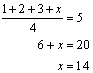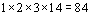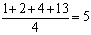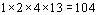# Extra Hard SAT Math Question – Mean Means

At the end of every SAT Math section, the test makers try to come up with an extremely difficult problem that will leave even the cleverest students scratching their heads. The really evil part, though, is that even these problems can be solved in under a minute without a calculator – if you know what to do. This means that once you “figure out the trick,” these difficult problems become easy. So, while those test makers are busy cackling with sadistic glee, let’s see if we can’t beat them at their own game.

Consider the following problem:

If the average (arithmetic mean) of four different positive integers is 5, then the least possible product of the four integers is:

A) 20

B) 84

C) 104

D) 480

E) 504

This one might seem like a hard nut to crack. After all, there are many possible sets of four distinct positive integers that have an average of 5. We could not possibly test them all one by one given the time constraints of the SAT. So how are we going to figure out which four integers are the ones that yield the smallest possible product?

Well, if we want the smallest product, then we want as many of the integers to be as small as possible. If the smallest of the three integers were equal to 1, then that would be great, since multiplying by one simply produces the same number and would thus not increase the product of the three numbers. Since the four integers must be distinct, we can make the second integer 2 so that it is also as small as possible, and by the same logic the third integer would be 3. All that remains is to determine the fourth integer, which we can find using algebra:The fourth integer is 14, and the product is:Logically, the answer must be 84, or choice B. If you are still feeling unsure, you could try a few other sets of four integers to see if their products are less than 84. What happens, for instance, when we increase the 3 to 4 and decrease the 14 to 13?